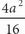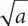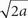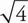# SAT Math Multiple Choice Question 139: Answer and Explanation

### Test Information

Question: 139

4. If a =and a is a nonzero integer, which of the following is equivalent to a ?

• A. 4a
• B. 4• C.• D. 2D The first step here is to simplify the equation and solve for a. Start by multiplying both sides by 16 to get 16a = 4a2. Divide both sides by 4 to get 4a = a2. Divide both sides by a to get 4 = a. This is now your target answer. Plug a = 4 into the values of a in the answer choices to determine which one matches 4. Choice (D) is the answer, since 2= 2= 2(2) = 4.